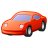# QlikView App Dev

Discussion Board for collaboration related to QlikView App Development.

Announcements
Join this live chat April 6, 10AM EST - QlikView to Qlik Sense REGISTER
cancel
Showing results for
Search instead for
Did you mean:Not applicable

## Handling Nulls

Hi,

I have a requirement wherein I have to diregard a particular division and display the sum of sales of the other Divisions.

In the sample application attached i am trying to acheive the same by disregarding the Division 'A'

These are the following approaches used by me:

Approach 1:

=sum({<Flag={1},
Division-={'A'}>}Sales)

This approach not only  excludes division A but it also excludes the NULL divisions,but all i want is to exclude the Division A and not the NULL divisions

Approach2:

=sum({<Flag={1},
Division={'*'}-{'A'}>}Sales)

This also gives me the result as in approach 1

Approach3:

=sum({<Flag={1}>
-<
Flag={1},Division={'A'}>}Sales)

This approach gives me the desired results.But I want to know if there is any built in function within qlikview that could be used to accomplish this requirement in a better way

1 Solution

Accepted SolutionsCreator III

In the script

NULLASVALUE *;

SET NULLVALUE = '<Unknown>';

LOAD Year,

Division,

Sales,

Flag

FROM

(ooxml, embedded labels, table is Sheet1);

Then the first equation should work....hopefully13 RepliesMaster III

Why dont you try removing null in your scriptNot applicable
Author

I need to show the Nulls also in the applicationMaster

Hi Anushree,

Use this one in Text object =Sum({\$<Flag={1},Division ={'A'}> + -<Division=-{}>}Sales)

I would suggest to create a new flag for Null value in Division field. So that we can control set analysis easily.

Regards,

SokkornCreator III

In the script

NULLASVALUE *;

SET NULLVALUE = '<Unknown>';

LOAD Year,

Division,

Sales,

Flag

FROM

(ooxml, embedded labels, table is Sheet1);

Then the first equation should work....hopefullyMVP & Luminary

Hi,

Try like this

NullAsValue Division;

Set NullValue = 'NULL' ;

Data:

LOAD

If(Len(Division) = 0, Null(), Division) AS Division, Flag, Sales, Year

INLINE [

Division, Flag, Sales, Year

A, 0, 50, 2012

A, 1, 10, 2011

A, 1, 30, 2013

B, 1, 40, 2013

B, 1, 50, 2011

C, 1, 20, 2011

C, 1, 50, 2013

C, 1, 80, 2012

D, 1, 70, 2012

D, 1, 80, 2013

E, 1, 40, 2011

E, 1, 50, 2012

, 1, 30, 2011

, 1, 60, 2012

, 1, 80, 2013

];

Now use this expression

=sum({<Flag={1},Division-={'A'}>}Sales)

Regards,

Jagan.Master

Hi,

My mistake in previous replied.

If you want to calculate sale amount where Division <> A and Division = Null Then we use =Sum({\$<Flag={1},Division -={'A'}> + -<Division=-{}>}Sales)

If you want to calculate sale amount where Division = A and Division = Null Then we use =Sum({\$<Flag={1},Division={'A'}> + -<Division=-{}>}Sales)

Regards,

SokkornSpecialist

your answer are clean and clear , very helpful !

anantNot applicable
Author

Hi Sokkorn,

The expression suggested by you works completely fine.But,the continuous usage of + and – in the expression,like + -<Division=-{}>}Sales seems a bit confusing to me so could you please explain the logic behind the same

Thanks & Regards,

Anushree ShettyMaster

Hi,

If we want to sum sale amount where division is null then this is the set analysis =Sum({\$-<Division=-{}>}Sales)

Now we try calculate other sale amount which division<>A, previously you used =Sum({<Flag={1},Division-={'A'}>}Sales)

Looking into your requirement, you need to sum sale amount base on division<>A and division=null

So I used =Sum({\$<Flag={1},Division -={'A'}> + -<Division=-{}>}Sales)

+ sign mean Union

- sign mean Exclusion

See attached file for details

Regards,

Sokkorn